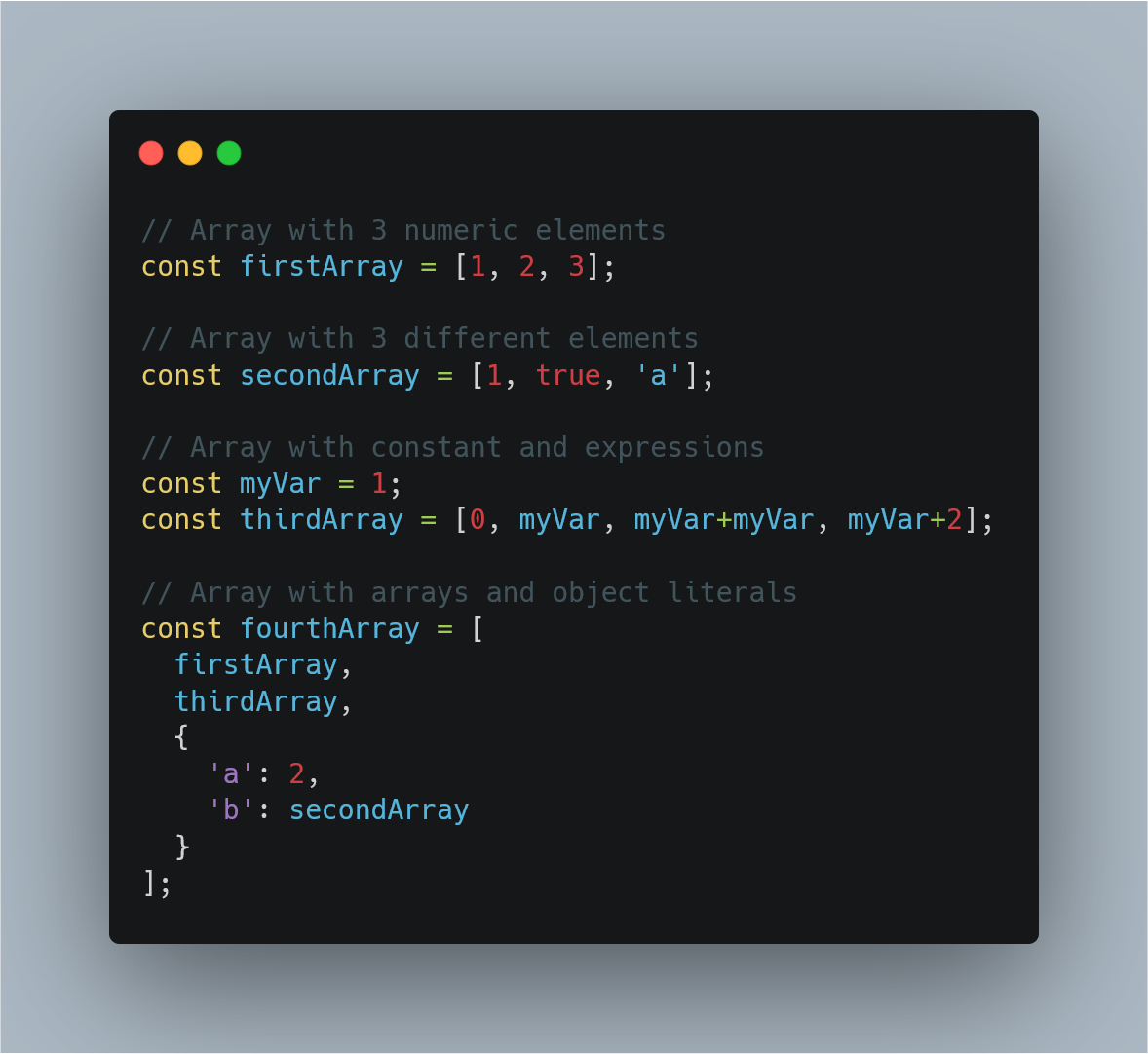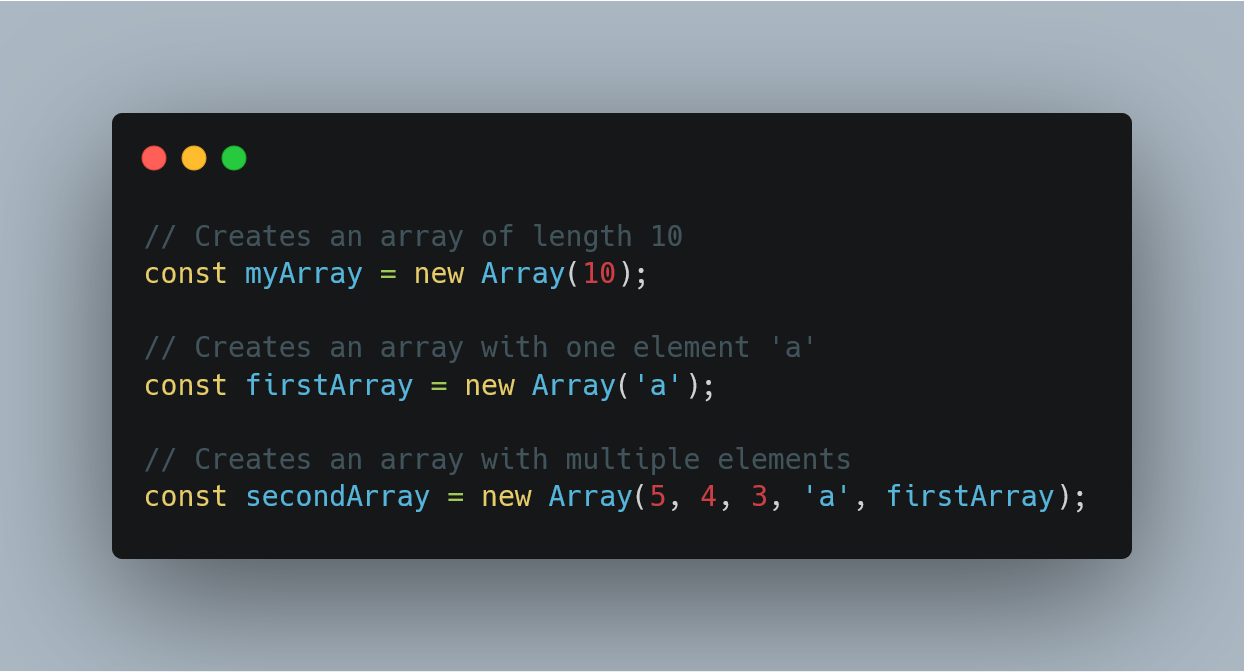1600481520

# Javascript array find: How to Find Element in Javascript

Javascript array find() is an inbuilt js function that returns the value of the first item in the Array that satisfies a provided testing function. Otherwise,  undefined will be returned. The array find() method returns the value of the first element in an array that passes a test of provided function.

If an Array find() method finds an item where the function returns a true value. Javascript find() returns the value of that array item immediately and does not check the remaining values of that Array.

### Javascript array find

Javascript Array.find() is the inbuilt function that is used to get a value of the first item in the Array that meets the provided condition. If you need an index of the found item in the Array, use the  findIndex(). If you need to find an index of the value, use Array  .prototype.indexOf(). If you need to find if the value exists in an array, use Array  .prototype.includes().

It checks all the items of the Array, and whichever the first item meets, the condition is going to print. If more than one item meets the condition, then the first item satisfying the requirement is returned. Suppose that you want to find the first odd number in the Array. The argument function checks whether an argument passed to it is an odd number or not.

Javascript find() function calls an argument function for every item of the Array.  The first odd number for which argument function returns true is reported by the find() function as the answer.

#javascript #javascript find #array.find

## Buddha Community1600481520

## Javascript array find: How to Find Element in Javascript

Javascript array find() is an inbuilt js function that returns the value of the first item in the Array that satisfies a provided testing function. Otherwise,  undefined will be returned. The array find() method returns the value of the first element in an array that passes a test of provided function.

If an Array find() method finds an item where the function returns a true value. Javascript find() returns the value of that array item immediately and does not check the remaining values of that Array.

### Javascript array find

Javascript Array.find() is the inbuilt function that is used to get a value of the first item in the Array that meets the provided condition. If you need an index of the found item in the Array, use the  findIndex(). If you need to find an index of the value, use Array  .prototype.indexOf(). If you need to find if the value exists in an array, use Array  .prototype.includes().

It checks all the items of the Array, and whichever the first item meets, the condition is going to print. If more than one item meets the condition, then the first item satisfying the requirement is returned. Suppose that you want to find the first odd number in the Array. The argument function checks whether an argument passed to it is an odd number or not.

Javascript find() function calls an argument function for every item of the Array.  The first odd number for which argument function returns true is reported by the find() function as the answer.

#javascript #javascript find #array.find1624388400

## JavaScript Arrays Tutorial. DO NOT MISS!!!

Learn JavaScript Arrays

📺 The video in this post was made by Programming with Mosh
The origin of the article: https://www.youtube.com/watch?v=oigfaZ5ApsM&list=PLTjRvDozrdlxEIuOBZkMAK5uiqp8rHUax&index=4
🔥 If you’re a beginner. I believe the article below will be useful to you ☞ What You Should Know Before Investing in Cryptocurrency - For Beginner
⭐ ⭐ ⭐The project is of interest to the community. Join to Get free ‘GEEK coin’ (GEEKCASH coin)!
Thanks for visiting and watching! Please don’t forget to leave a like, comment and share!

#arrays #javascript #javascript arrays #javascript arrays tutorial1602154740

## Fill and Filter in Array in JavaScript

By the word Array methods, I mean the inbuilt array functions, which might be helpful for us in so many ways. So why not just explore and make use of them, to boost our productivity.

Let’s see them together one by one with some amazing examples.

### Array.fill():

The `_fill()_` method changes all elements in an array to a static value, from a start index (default `_0_`) to an end index (default `_array.length_`). It returns the modified array.

In simple words, it’s gonna fill the elements of the array with whatever sets of params, you pass in it. Mostly we pass three params, each param stands with some meaning. The first param value: what value you want to fill, second value: start range of index(inclusive), and third value: end range of index(exclusive). Imagine you are going to apply this method on some date, so that how its gonna look like eg: array.fill(‘Some date’, start date, end date).

``````NOTE: Start range is inclusive and end range is exclusive.
``````

Let’s understand this in the below example-

``````//declare array
var testArray = [2,4,6,8,10,12,14];

console.log(testArray.fill("A"));
``````

When you run this code, you gonna see all the elements of `testArray` will be replaced by `'A'` like `[“A”,"A","A","A","A","A","A"]`.

#javascript-tips #array-methods #javascript-development #javascript #arrays1625988600

## JavaScript Array Methods #4: Array .find() Method

The Javascript Array .find() method is used to get the value of the first element in the array that satisfies the provided condition in a callback function. It checks all the elements of the array and whichever first element satisfies the condition is going to print.

The function and syntax of find() are very much like the Array .filter() method, except it, only returns a single element. Another difference is when nothing is found, this method returns a value of undefined.

Syntax:
array.find(function(currentValue, index, arr), thisValue)

▶ #1: Array .map() Method | JavaScript Array Methods

▶ #2: Array .forEach() Method | JavaScript Array Methods

▶ #3: Array .filter() Method | JavaScript Array Methods

▶ JavaScript Array Methods

▶ Vue 2 Basics Beginner Tutorial

▶ Vue.js 2 Components, Beginners tutorial

▶ Vuex tutorial (Vue.js state management)

▶ Vue.js 3 Tutorial

Support my work:

2. On Patreon: https://www.patreon.com/qirolab

𝐃𝐢𝐠𝐢𝐭𝐚𝐥𝐎𝐜𝐞𝐚𝐧 𝐑𝐞𝐟𝐞𝐫𝐫𝐚𝐥
https://m.do.co/c/e740238537d0

𝐅𝐚𝐜𝐞𝐛𝐨𝐨𝐤: https://fb.com/qirolab

#javascript #array #find1597470780

## Introduction

Arrays are a structure common to all programming languages so knowing what they are and having a firm grasp on what you’re able to accomplish with Arrays will take you a long way in your journey as a software developer. The code examples I share in this post will be in JavaScript but the concepts are common among all languages. What you learn here can easily be translated to any other language you work with.

In this post I’ll be covering how to perform the create, read update and delete operations using arrays, some common functions that come with the Array prototype and also how to implement them.

## What is an Array

Before we jump into the juicy bits of Arrays, lets quickly gloss over what they are. Arrays

• are a fundamental data type in JavaScript
• are an ordered collection of values called **elements **that are stored at and accessed via an index
• are untyped, meaning that the elements of an array could be of different types. This allows us to create complex arrays such as an array of objects or even an array of arrays (multidimensional arrays)
• can have elements that are constants or expressions
• have a property called length that tells you the number of elements in the array
• inherit properties from `Array.prototype` that includes a wide variety useful functions that can be called from arrays or `array-like` objects

## CRUD operations using Arrays

If you’re not familiar with the term CRUD it stands for Create, Read, Update and Delete. In this section we’ll go through each one of these operations and cover different ways you can perform each one.

### Creating Arrays

There are several ways you can create an Array but the most common ways are by using

• the Array literal syntax
• the Array constructor i.e. `new Array()`

Lets take a look at each one with examples

### Array literal

The array literal is the most common way of creating an array. It uses the square brackets as a notion of a container followed by comma separated values inside the square brackets. The following examples show how to use the array literal syntax and how arrays are untyped i.e. can contain elements of different types.Examples of untyped arrays in JavaScript created with the array literal syntax.

### Array constructor

Another way to create an array is through the Array constructor.

``````const myArray = new Array();
``````

Using the Array constructor, as shown above, is the same as creating an array with the array literal syntax. i.e.

``````// The following two lines behave exactly the same way i.e. both create an empty arrays

const myArray = new Array();
const myOtherArray = [];
``````

The array constructor, however, is able to receive arguments that allow it to behave in different ways depending on the number and type of arguments passed to it.

• You can pass a single numeric argument which creates an array of the specified length. This option is mostly used when you know how many elements you’ll be placing in the array
``````const myArray = new Array(5);
``````

Note: If you want to define the array with a specified size, as shown above, the argument passed must be a numeric value. Any other type would be considered as the first element that’ll be placed in the array.

• Or you can pass two or more arguments or a non-numeric argument to place the values inside the array. This works the same way as shown in the array literal examples.Examples of arrays created by using the Array constructor in JavaScript

As stated earlier, these two ways are the most common ways of creating arrays that you’ll see and use 99% of the time. There are a few other ways but we won’t dive deep into how they work. They are

• the spread operator `const someArray = […someOtherArray]`
• the static method `Array.of()`
• and the static method `Array.from()`

#javascript #web-development #javascript-tips #javascript-development #javascript-arrays #sql